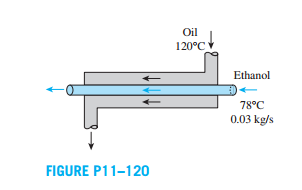Create an Account

Home / Questions / Ethanol is vaporized at 78°C hfg 846 kJkg in a double pipe parallel flow heat exchanger at...

Ethanol is vaporized at 78°C hfg 846 kJkg in a double pipe parallel flow heat exchanger at a rate of 0 03 kg s by hot oil

Ethanol is vaporized at 78°C (hfg =846 kJ/kg) in a double-pipe parallel-flow heat exchanger at a rate of 0.03 kg/s by hot oil (cp = 2200 J/kg.K) that enters at 120°C. If the heat transfer surface area and the overall heat transfer coefficients are 6.2 m2 and 320 W/m2. K, respectively, determine the outlet temperature and the mass flow rate of oil using (a) the LMTD method and (b) the ε- NTU method.Jul 23 2020 View more View LessSubscribe To Get Solution